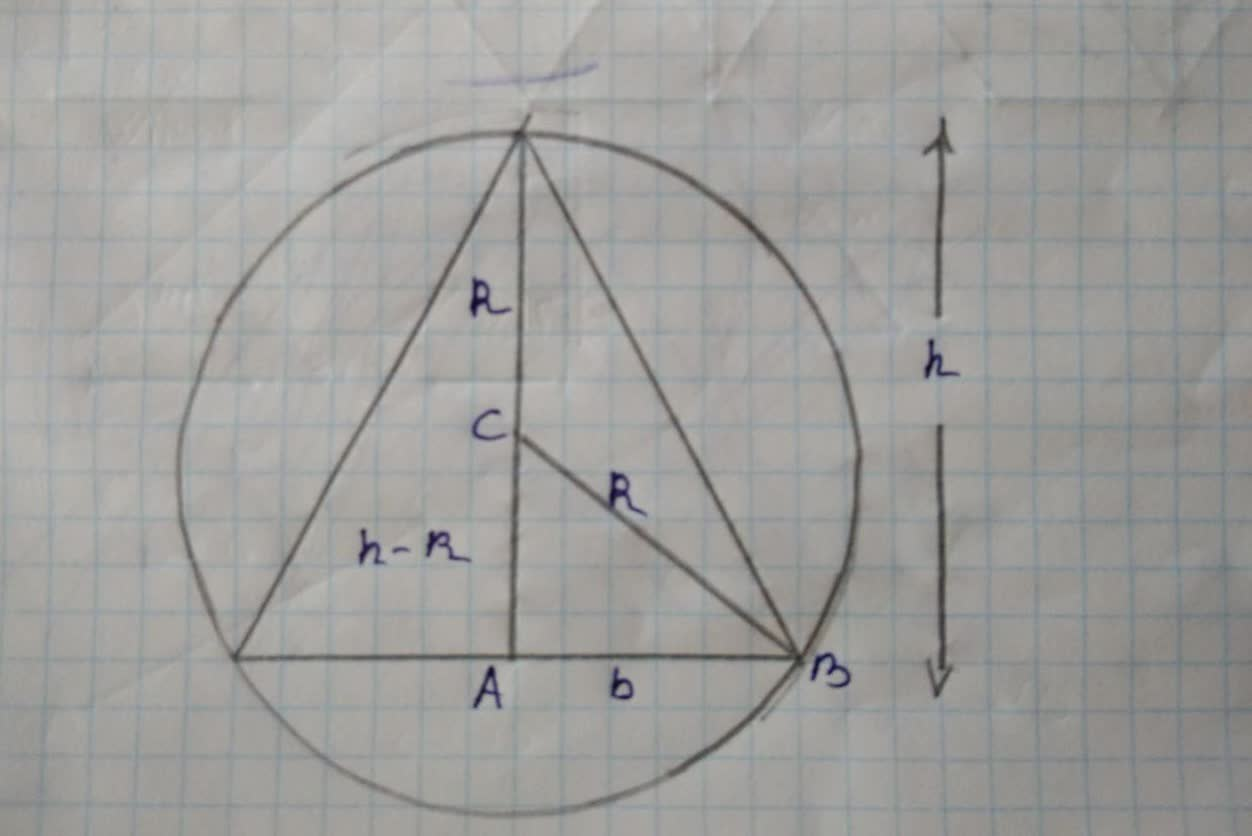# Find the area of the largest isosceles triangle that canGregory Emery 2022-01-10 Answered
Find the area of the largest isosceles triangle that can be inscribed in a circle of radius 6.
a) Solve by writing the area as a function of h.
b) Solve by writing the area as a function of a.
c) Identify the type of triangle of maximum area.
You can still ask an expert for help

• Questions are typically answered in as fast as 30 minutes

Solve your problem for the price of one coffee

• Math expert for every subject
• Pay only if we can solve ittemnimam2

See the figure aboveUsing Pythagoras theorem, we can write
$b=\sqrt{{R}^{2}-{\left(h-R\right)}^{2}}$
$b=\sqrt{{R}^{2}-\left(h-{R}^{2}-2Rh\right)}$
$b=\sqrt{2Rh-{h}^{2}}$
Length of the Base $=2b=2\sqrt{2Rh-{h}^{2}}$
In the given problem, radius of the circle is 6. Therefore
Length of the Base $=2\sqrt{12Rh-{h}^{2}}$
Area of a triangle
Area of a triangle$=\frac{1}{2}\cdot 2\sqrt{12h-{h}^{2}}×h$
$A\left(h\right)=h\sqrt{12Rh-{h}^{2}}$
Differentiate
${A}^{\prime }\left(h\right)=\sqrt{12Rh-{h}^{2}}+\frac{h\left(12-2h\right)}{2\sqrt{12h-{h}^{2}}}$
${A}^{\prime }\left(h\right)=\sqrt{12Rh-{h}^{2}}+\frac{6h-{h}^{2}}{2\sqrt{12h-{h}^{2}}}$
Equate A’(h) to 0
$\sqrt{12Rh-{h}^{2}}+\frac{6h-{h}^{2}}{2\sqrt{12h-{h}^{2}}}=0$
Multiply throughout by $\sqrt{12Rh-{h}^{2}}$
$\left({\left(12h-h\right)}^{2}+\left(6h={h}^{2}\right)=0$
$18h—2{h}^{2}=0$
$\mid {n}^{2}=9$
$h=3$
Area cannot be minimum when h = 3, because minimum area is 0.

###### Not exactly what you’re looking for?deginasiba
For solving (b), we can find the total height $\left({h}_{T}\right)$ of the isosceles triangle by adding the value for h found in (a) to the other height 6:
${h}_{T}=6+3=9$.
So, we can say that the total height of the triangle is 9 units. Then we can find the base:
$b=\sqrt{36-{3}^{2}}=\sqrt{36-9}=\sqrt{27}=3\sqrt{3}$,
We can then divide the base by 2 to get the length of the base for half the triangle (by the AAS.
Similar Triangles Theorem) to get the base to be $\frac{3\sqrt{3}}{2}$ , We can now focus on the right half of the
triangle divided by the dotted line going through the vertex point of the circle, We can tell that the
dotted line through the vertex makes a right angle. Thus, we ean use Trigonometry to solve for a.
So,
$a=\mathrm{tan}\frac{opposite}{adjacent}=\mathrm{tan}\frac{\frac{3\sqrt{3}}{2}}{9}=\mathrm{tan}\frac{27\sqrt{3}}{2}$.
Since we have found what a is, we can find the function of it with respect to a:
$\phantom{\rule{0ex}{0ex}}tan\left(\begin{array}{c}\frac{\left(6+h\right)\left(\sqrt{36+{h}^{2}\right)}}{2}\end{array}\right)$.
or as an incorporated function:
$\frac{1}{2}bh=\frac{1}{2}\left(6\mathrm{sin}a\right)\left(12\mathrm{cos}a\right)=36\mathrm{sin}a\mathrm{cos}a$.
And we are done with (b)
For (c), By the Isoperimetric Theorem, it states
Theorem: Among all triangles inscribed in a given circle, the equilateral one has the largest area.
Therefore, the equilateral triangle has the maximum area.